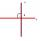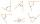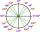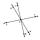Slope

What is the slope of a line with an inclination -221°?

Result

k =  -0.87

Solution:Leave us a comment of example and its solution (i.e. if it is still somewhat unclear...):Be the first to comment!To solve this example are needed these knowledge from mathematics:

For Basic calculations in analytic geometry is helpful line slope calculator. From coordinates of two points in the plane it calculate slope, normal and parametric line equation(s), slope, directional angle, direction vector, the length of segment, intersections the coordinate axes etc. See also our right triangle calculator. Most natural application of trigonometry and trigonometric functions is a calculation of the triangles. Common and less common calculations of different types of triangles offers our triangle calculator. Word trigonometry comes from Greek and literally means triangle calculation.

Next similar examples:

1. SlopeFind the slope of the line: x=t and y=1+t.
2. V - slopeThe slope of the line whose equation is -3x -9 = 0 is
3. PerpendicularDetermine the slope of the line perpendicular to the line p: y = -x +4.
4. Supplementary anglesOne of the supplementary angles are three times larger than the other. What size is larger of supplementary angles?
5. ReflectorCircular reflector throws light cone with a vertex angle 49° and is on 33 m height tower. The axis of the light beam has with the axis of the tower angle 30°. What is the maximum length of the illuminated horizontal plane?What is greater angle? -0.7 radians or -0.7π radians?
7. Theorem proveWe want to prove the sentence: If the natural number n is divisible by six, then n is divisible by three. From what assumption we started?
8. The angle of linesCalculate the angle of two lines y=x-21 and y=-2x+14
9. LineIt is true that the lines that do not intersect are parallel?
10. LineLine p passing through A[-10, 6] and has direction vector v=(3, 2). Is point B[7, 30] on the line p?
11. High wallI have a wall 2m high. I need a 15 degree angle (upward) to second wall 4 meters away. How high must the second wall?
12. TreeHow tall is the tree that observed in the visual angle of 52°? If I stand 5 m from the tree and eyes are two meters above the ground.
13. CosineThe point (8, 6) is on the terminal side of angle θ. cos θ = ?
14. MapleMaple peak is visible from a distance 3 m from the trunk from a height of 1.8 m at angle 62°. Determine the height of the maple.
15. LiftThe largest angle at which the lift rises is 16°31'. Give climb angle in permille.
16. DistanceWha is the distance between the origin and the point (18; 22)?
17. Reference angleFind the reference angle of each angle: